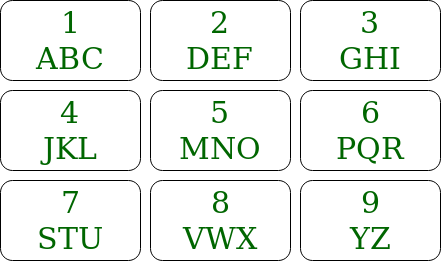Related Articles

# Java ArrayList to print all possible words from phone digits

• Difficulty Level : Hard
• Last Updated : 23 Jul, 2021

Given a keypad of a mobile, and keys that need to be pressed, the task is to print all the words which are possible to generate by pressing these numbers.Examples:

```Input: str = "12"
Output: [ad, bd, cd, ae, be, ce, af, bf, cf]
Explanation: The characters that can be formed
by pressing 1 is a, b, c and by pressing 2 characters
d, e, f can be formed.
So all the words will be a combination where first
character belongs to a, b, c and 2nd character belongs
to d, e, f

Input: str = "4"
Output: [j, k, l]
Explanation: The characters that can be formed
by pressing 4 is j, k, l```

Method 1: Another approach is discussed here Print all possible words from phone digits
Method 2:
Approach: The approach is slightly different from the approach in the other article. Suppose there are n keys which are pressed (a1 a2 a3 ..an). Find all the words that can be formed using (a2 a3 ..an). Suppose 3 characters can be generated by pressing a1 then for every character concatenate the character before all the words and insert them to the list.
For Example:

If the keypress is 12
The characters that can be formed by pressing 1 is a, b, c and by pressing 2 characters d, e, f can be formed.
So all the words that can be formed using 2 are [d, e, f]
So now concatenate ‘a’ with all words returned so, the list is [ad, ae, af] similarly concatenate b and c. So the list becomes [ad, ae, af, bd, be, bf, cd, ce, cf].

Algorithm:

1. Write a recursive function that accepts key press string and returns all the words that can be formed in an Array list.
2. If the length of the given string is 0 then return Arraylist containing empty string.
3. Else recursively call the function with a string except the first character of original string, i.e string containing all the characters from index 1 to n-1. and store the arraylist returned, list and create a new arraylist ans
4. Get the character set of the first character of original string, CSet
5. For every word of the list run a loop through the Cset and concatenate the character of Cset infront of the word of list and insert them in the ans arraylist.
6. Return the arraylist, ans.

Implementation:

## Java

 `// Java implementation of the approach``import` `java.util.ArrayList;` `public` `class` `GFG {` `    ``// String array to store keypad characters``    ``static` `final` `String codes[]``        ``= { ``" "``, ``"abc"``, ``"def"``,``            ``"ghi"``, ``"jkl"``, ``"mno"``,``            ``"pqr"``, ``"stu"``, ``"vwx"``,``            ``"yz"` `};` `    ``// Function that returns an Arraylist``    ``// which contains all the generated words``    ``public` `static` `ArrayList printKeyWords(String str)``    ``{` `        ``// If str is empty``        ``if` `(str.length() == ``0``) {``            ``ArrayList baseRes = ``new` `ArrayList<>();``            ``baseRes.add(``""``);` `            ``// Return an Arraylist containing``            ``// empty string``            ``return` `baseRes;``        ``}` `        ``// First character of str``        ``char` `ch = str.charAt(``0``);` `        ``// Rest of the characters of str``        ``String restStr = str.substring(``1``);` `        ``ArrayList prevRes = printKeyWords(restStr);``        ``ArrayList Res = ``new` `ArrayList<>();` `        ``String code = codes[ch - ``'0'``];` `        ``for` `(String val : prevRes) {` `            ``for` `(``int` `i = ``0``; i < code.length(); i++) {``                ``Res.add(code.charAt(i) + val);``            ``}``        ``}``        ``return` `Res;``    ``}` `    ``// Driver code``    ``public` `static` `void` `main(String[] args)``    ``{``        ``String str = ``"23"``;` `        ``// Print all the possible words``        ``System.out.println(printKeyWords(str));``    ``}``}`
Output:
`[dg, eg, fg, dh, eh, fh, di, ei, fi]`

Complexity Analysis:

• Time Complexity: O(3n).
Though the recursive function runs n times. But the size of the arraylist grows exponentially. So there will be around 3n elements in the arraylist. Therefore, traversing them will take 3n time.
• Space Complexity:O(3n).
Space required to store all words is O(3n). As there will be around 3n words in the output.My Personal Notes arrow_drop_up